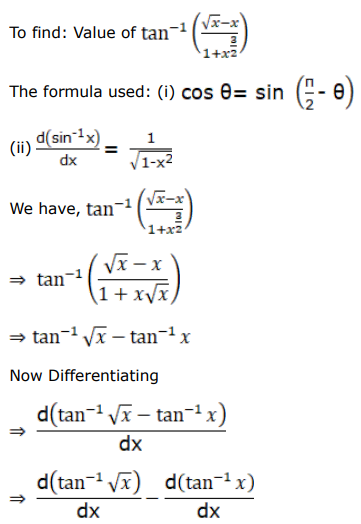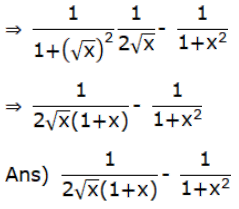# Differentiate each of the following w.r.tQuestion:

Differentiate each of the following w.r.t $x$ :

$\tan ^{-1}\left\{\frac{\sqrt{x}-x}{1+x^{3 / 2}}\right\}$

Solution: